# Nyquist Diagram from Data

roam

## Homework Statement

I'm trying to make a Nyquist diagram based on experimental data obtained of the gain and phase of a high-pass RC filter which has the transfer function:

##\frac{V_{out}}{V_{in}}=\frac{R}{R+1/(j \omega C)}=\frac{1}{1-j\omega_0/\omega}##

Here are the superimposed experimental and theoretical Bode plots: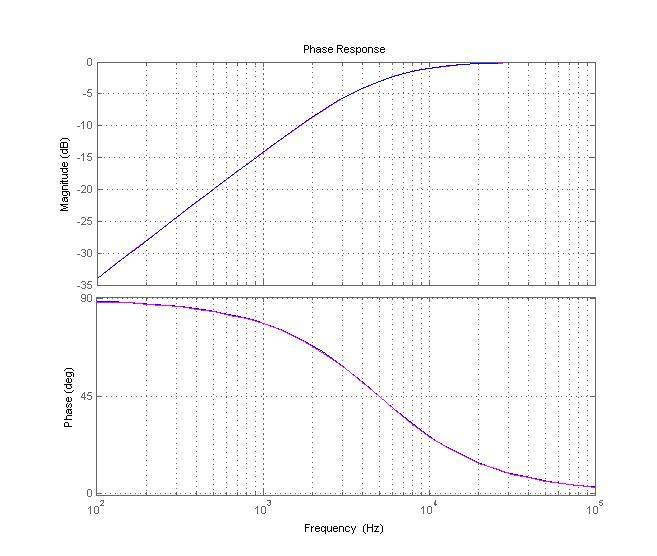And here is the theoretical Nyquist plot I made by using the equation above with Matlab's nyquist() function: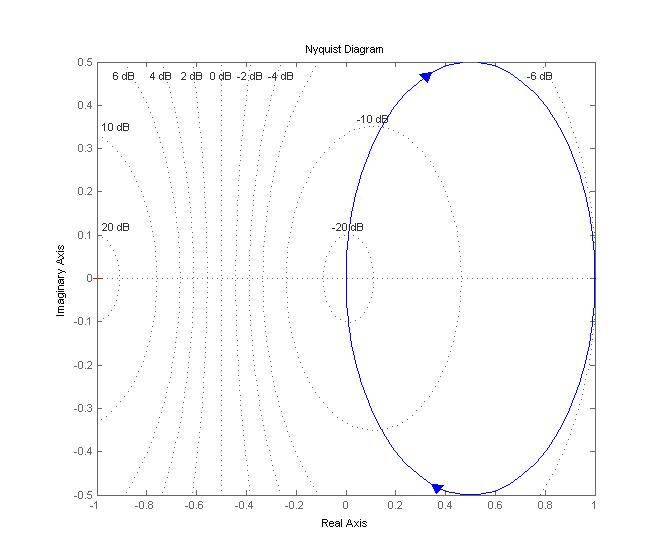So how can I make an experimental Nyquist plot (using the two sets of data obtained on amplitude and phase of the filter)?

## The Attempt at a Solution

Unfortunately I couldn't get any help with this problem so far and I haven't found anything relevant online.

So, I have 3 vectors containing the collected data (gain in dB, phase in degrees, frequency in Hertz). How can I plot the Nyquist diagram using the data and NOT the transfer function?Is there a simple way that Matlab can turn my data into a Nyquist plot? Or do I need to do everything manually? If it has to be done manually, then how should I approach this?

Any explanation, sample code, or perhaps links would be greatly appreciated.

P. S. I've attached my data just in case.

#### Attachments

• Data.txt
1.2 KB · Views: 522

milesyoung
If your system doesn't have any zeros or poles at the origin, then its Nyquist diagram is just its Bode plot in polar form with its complex conjugate superimposed on top of it, so if you have your gain (in linear magnitude) and phase (in radians) in a couple of vectors, then one half of the Nyquist diagram is simply given by:

Code:
z = gain.*exp(j*phase);
plot(z)

You can complete it using:
Code:
plot([z;conj(z(end:-1:1))])

You'll have to mentally add the direction, but that should be simple enough.

•1 person
Homework Helper
Gold Member

## Homework Statement

I'm trying to make a Nyquist diagram based on experimental data obtained of the gain and phase of a high-pass RC filter which has the transfer function:

##\frac{V_{out}}{V_{in}}=\frac{R}{R+1/(j \omega C)}=\frac{1}{1-j\omega_0/\omega}##

For starters, this is not in the correct form. You need to rewite your transfer function so ω appears in the denominator as a term in ω, not 1/ω. At least, that is the convention. Whether you can proceed the way you did is questionable to me.

milesyoung
For starters, this is not in the correct form. You need to rewite your transfer function so ω appears in the denominator as a term in ω, not 1/ω. At least, that is the convention. Whether you can proceed the way you did is questionable to me.
I assume you're talking about the form that shows break frequencies for use in, for instance, drawing Bode plots using asymptotic approximations. That form isn't necessarily "correct", or even good, for drawing Nyquist diagrams. You can often find a much more convenient polar form of the transfer function for these, i.e. it's not always better to draw Bode plots first and then use them to sketch the Nyquist diagram (Edit: Just to elaborate, straight lines in Bode plots aren't that great when drawing in polar form).

In any case, the OP's question was about plotting a Nyquist diagram from a data set, not a transfer function.

Last edited:
roam
Thank you for the helpful post Miles. But how would you modify the code if the transfer function contains a zero/pole at the origin?

For instance I tried your method for an LC resonant circuit (the notch filter) which had a transfer function of:

##\frac{V_{out}}{V_{in}} = \frac{R_2}{R_2+z_p}##

with ##z_p = \frac{R_p}{1+jR_p(\omega C -\frac{1}{\omega L})}##

It simplifies to ##\therefore \frac{R_2 s}{R_2 s + R_ps + (1/C) - (1/L)}## (s=jω)

Clearly it has a zero at the origin. In fact here is the diagram I got when I tried using that code (magenta is the experimental, blue is the theoretical):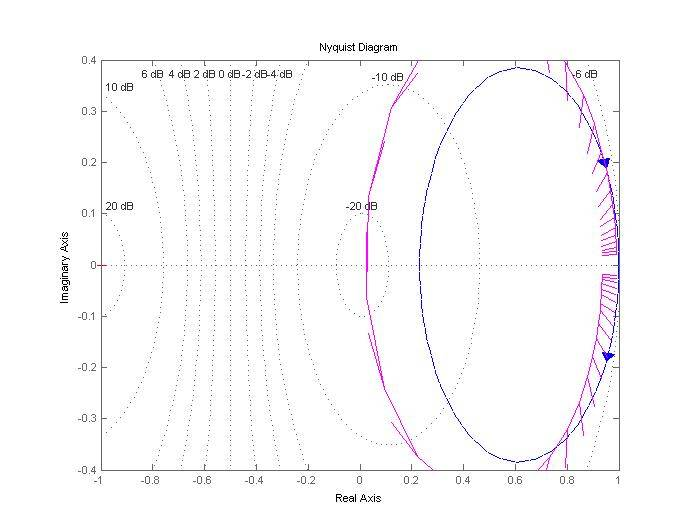I have the same problem with plotting Nyquist diagram of LC bandpass filters. I'm not quite sure why that happens and how to fix it.#### Attachments

• Notch.txt
625 bytes · Views: 470
roam
Similarly, I tried to make a Nyquist diagram of a bandpass filter with transfer function:

##\frac{R_1}{R_1 + R_s + sL+1/sC}## (R1=83Ω, L=10mH, C=22nF, Rs=20Ω). Once again it has a zero at the origin. This is what I got using experimental data (not the TF):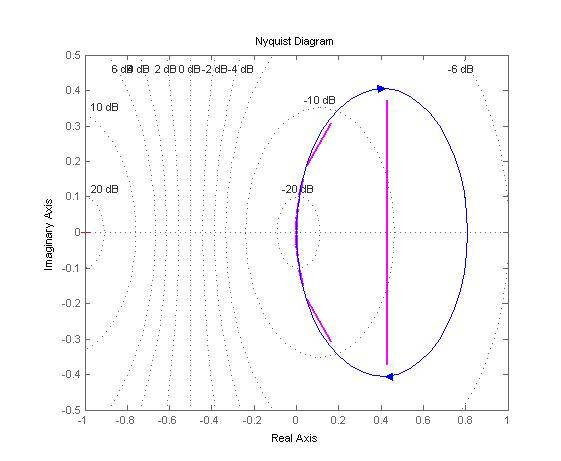(Magenta is the experimental one)

What is causing the straight line at the center, and how can we get rid of it?

#### Attachments

• bandpass.txt
725 bytes · Views: 466
milesyoung
But how would you modify the code if the transfer function contains a zero/pole at the origin?
You don't have to modify it, you just have to remember that the frequency response of the system only gives part of its Nyquist diagram. That fact is more important when you have a zero or pole at the origin, because you then have to change the Nyquist path to "go around" that zero or pole, which either gives an arc around the origin or out at infinity, respectively, in your Nyquist diagram:
What does the Nyquist plot look like for a system with poles at the origin?

I'm not exactly sure what you're doing in those plots - mine look very different. Maybe you could post your source code? (use CODE tags).

They are, however, going to look a bit odd for the data sets you've provided. You only have a couple of data points at the resonance frequency of your filters, which isn't going to make for a great Bode plot or Nyquist diagram. You should have a higher resolution around where the magnitude/phase starts changing rapidly with frequency. 'plot' in MATLAB does linear interpolation between points, so you can get some odd looking graphs if you're not careful.

On another note, when uploading data sets, it's a good idea to use a standard format, like delimiter-separated values. MATLAB, for instance, can export workspace variables as comma-separated values in an ASCII file using the command 'csvwrite' (not with the greatest accuracy, but still).

roam
Here's my source code for the bandpass filter:

Code:
gain=10.^((1/20).*[-59.047 -57.164 etc...]);

% Experimental Nyquist plot

phase=0.0174532.*[90.029 89.812 etc...];

plot([z;conj(z(end:-1:1))], 'm', 'LineWidth', 2);

% Theoretical plot for comparison

hold on

R1=83.02;
Rs=19.49;

s = tf('s');
n=Rs*(1+(((s*L)/Rs)+(1/(s*Rs*C))));

sys = R1/(R1+n);
nyquist(sys, '--')
grid;

For phase and amplitude I just used the data attached to the previous post (the actual data are in degrees and dB respectively so they are multiplied by a conversion factor).

Yes, I did use separated values when entering the data set. I've attached the experimental Bode plot (gain being on the vertical axis).

So how is your plot different from mine?Did you use a different code??

P.S. The transfer function for this filter was:

##T= \frac{R_1}{R_1 + z_s}## where ##z_s=R_s \left[ 1+j(\frac{\omega L}{R_s}) - \frac{1}{\omega R_s C} \right]##

The series resistance is ##20 \Omega## and ##R_1=82 \Omega##, ##L= 10 mH##, ##C=22 nF##.

#### Attachments

Last edited:
milesyoung
You've written MATLAB commands directly into your 'Data.txt' file. When I enter those commands in my MATLAB workspace, I get gain and phase column vectors. In your other files, they're row vectors. This is why I suggested you use, for instance, 'csvwrite' to export data instead.

This:
Code:
plot([z;conj(z(end:-1:1))])
is meant to work with column vectors (since it just simply appends one column vector in reverse order to another). If you try using it for row vectors, you'll give 'plot' a 2 by something complex matrix, which it will try to plot column-wise.

For row vectors, you can use this instead:
Code:
plot([z conj(z(end:-1:1))])

This works for both cases:
Code:
plot(z)
hold on
plot(conj(z))

But again, these are still going to look terrible since you have so few data points around the resonance frequencies of your filters.

roam
Thanks for the remark, I made the correction to all my plots.

But are you sure this is because there is not have enough data points around resonance? Because for the previous RC circuits, I had the same amount of data, and yet the experimental Nyquist diagram closely matched the theory!

Is there any way to somehow smooth out the diagram a little? With the correction this is how the plots look like so far:

Band-pass: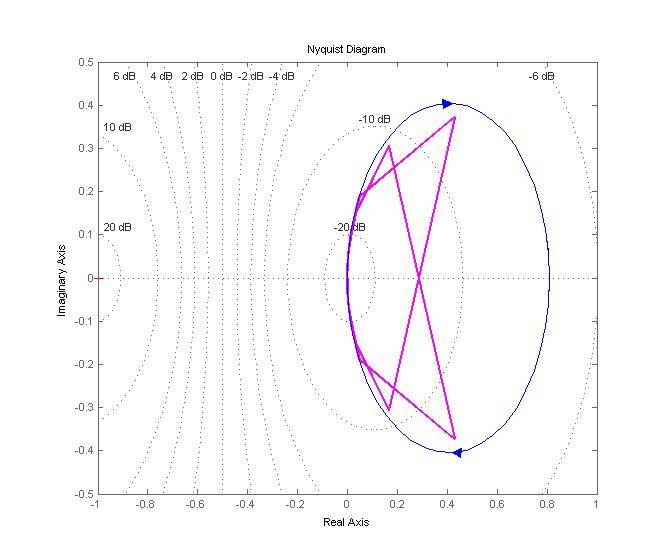Notch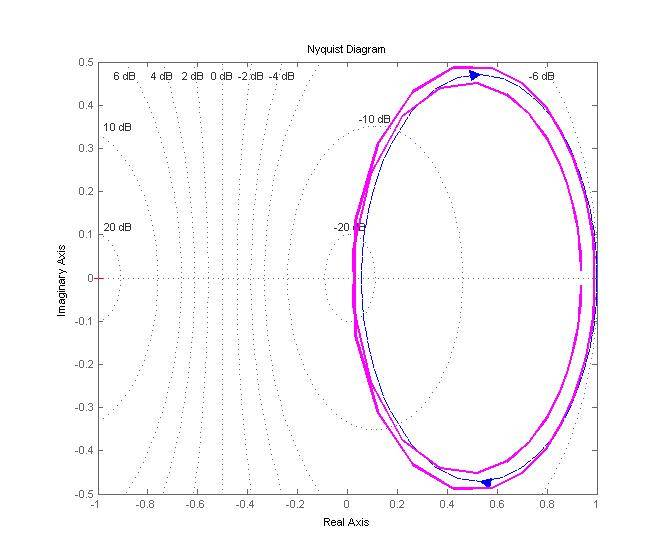I don't see why there's a double line in the last one...

milesyoung
As you can tell from your first diagram, there's only so much fidelity you can get using linear interpolation with so few data points around the resonance frequency. The problem is that the phase changes rapidly (at high magnitude) from something positive to something negative in a few data points, so you get those straight lines darting around and connecting those few points.

It's not that big of a deal for a notch filter, however, since that phase transition occurs at very low magnitude, so it really doesn't alter the overall shape of the Nyquist locus.

Compare how rapidly the magnitude/phase changes with frequency for your RC-circuit and your LC-circuits. You don't have the same amount of data around where it matters!

If you don't want to measure any more data, then it might be an option to just superimpose your data on a Bode plot of a filter with a transfer function of the right form, but where you can adjust the parameters until "it fits". Just try and eyeball it for now - I don't think it's worth getting into system identification just for these simple systems.

milesyoung
I don't see why there's a double line in the last one...
It's because, for the ideal filter, "one half" of the Nyquist diagram is already an ellipse in the complex plane. When you superimpose its complex conjugate, you do so right on top of the locus that's already there.

For your data set, that second "go around" isn't going to fit perfectly due to measurement errors and what not, so you get to see the whole shebang.

roam
Thank you for the explanation (the system doesn't allow me to thank you twice). It makes a lot more sense now.

milesyoung
You're very welcome.

I should probably have mentioned something in my first post: 'Phase' in the complex plane is synonymous with 'angle'. In your original post, can you see how that Bode plot traces out the upper half of the Nyquist diagram?

A magnitude and phase pair in the Bode plot gives a point in the complex plane with the same magnitude and phase (angle).

•roam